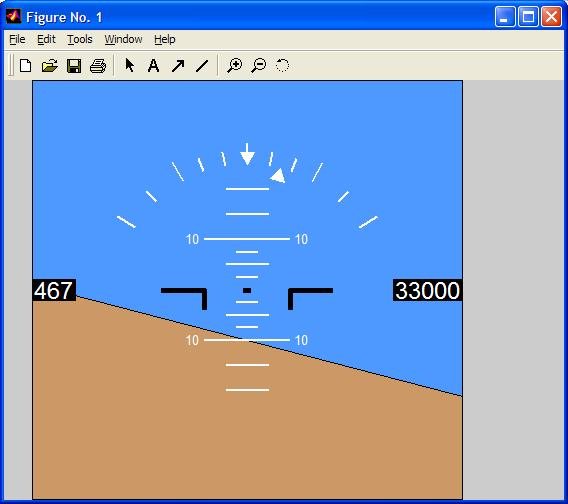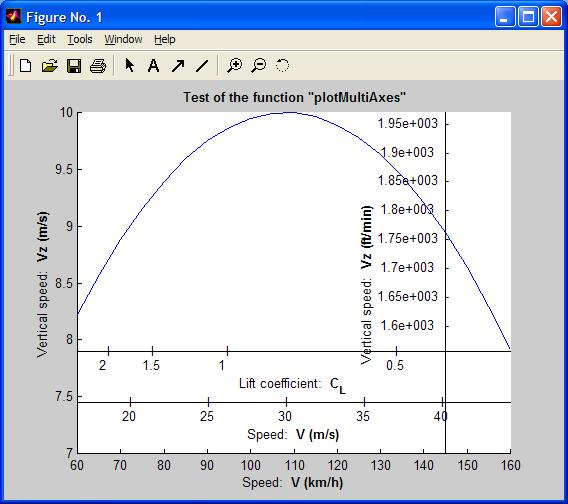I made these files for the fun or for my own studies. If it could help you ...Here it is !

There are several Matlab functions:

 Artificial Horizon instrument Display : "functionDisplay.m" Matlab functionLanguage: Matlab function Inputs: Pitch angle (radians) - Assiette Bank angle (radians) - G¯te Altitude (meters) - Altitude True Air Speed (m/s) - TAS To call the function, you have to do (for example): ``` Gite = -15*pi/180; % radians Assiette = 10*pi/180; % radians altitude = 33000*0.3048; % m TAS = 240; % m/s SimulateurInitialisation displaymodifie=functionDisplay(Assiette,Gite,altitude,TAS)``` Outputs: As you can see on the chart at the left, the output is a figure (with its handle properties) in which the Artificial Horizon is drawn at the flight position that you specified. What does this function do? This function draws the Artificial Horizon on Matlab figure. Altitude is indicated in feet on the right. True Air Speed (kt) is shown on the left. How does this function do it? It uses the Matlab function "patch" and its handle properties. Why does this function do it? I did it for the fun, but it could be used in output simulator... Some improvements should be done with the display of Course angle and Vertical speed Vz. download it for free

 Dessine-moi un avion... : "DessineMoiUnB747.m" Matlab functionWould you like to draw an aircraft in 3 dimensions with Matlab ? I give you this oportunity with the "DessineMoiUnB747.m" function. This allows you to draw a B747 in three dimensions in accordance with the inputs you gave: course angle, pitch angle, bank angle, altitude. Language: Matlab function Inputs: Course angle (radians) - Cap Pitch angle (radians) - Assiette Bank angle (radians) - G¯te Altitude (meters) - Altitude To call the function, you have to do (for example): ``` Gite = -5 *pi/180; Assiette = 12 *pi/180; Cap = 0 *pi/180; altitude = 15; DessineMoiUnB747(Cap,Assiette,Gite,altitude)``` Outputs: As you can see on the chart at the left, the output is a figure (with its handle properties) in which the B747 is drawn at the flight position that you specified. What does this function do? This function draws a B747 in a 3D Matlab figure. How does this function do it? It uses the Matlab function "patch('Vertices',vertices,'Faces',faces)" to draw the B747. Rotation matrices allow to put the B747 on the flight attitude you want. Why does this function do it? This function can be used to illustrate some reports; to show airplane in a specific flight attitude. This function can be used to make flight dynamics animations. For example, it could be usefull to show to the students how the airplane moves in dutch roll, or during a phugo´d... You only have to give the angles and altitude evolution (from solving dynamic of flight equations) to the function DessineMoiUnB747.m, and you could watch the airplane moves. ``` % Example to make the airplane moves for(i=[0:1:15 15:-1:0]), clf, DessineMoiUnB747(0,i*pi/180,0,37); pause(0.0001) end ``` download it for free

 UIUC Airfoil data importation : "functionReadAirfoilData.m" Matlab functionLanguage: Matlab function Inputs: Airfoil definition data file (it only reads the Lednicer format files) that you can download from Michael Selig's web site (UIUC). The Input is the name file you want to study. To call the function, you have to do (for example): ``` file='b737a.dat'; Data = functionReadAirfoilData(file);``` Outputs: The produced Output is a matlab structure: ```% Data.title = 'BOEING 737 ROOT AIRFOIL' % first file line: airfoil name % Data.x_upper = [23x1 double] % undimensionless upper airfoil abscisse. % Data.y_upper = [23x1 double] % undimensionless upper airfoil data: % % y_upper = y_upper(x_upper) % Data.x_lower = [23x1 double] % undimensionless lower airfoil abscisse. % Data.y_lower = [23x1 double] % undimensionless lower airfoil data: % % y_lower = y_lower(x_lower) % Data.thickness_chord = 0.1537 % determined by splining``` What does this function do? This function allows you to import airfoil definition from UIUC web site. How does this function do it? It uses the Matlab function 'fopen' and creates a structure in output. Why does this function do it? This function can be used to work on profils studies with matlab (to determine profils characteristics, Cmo, Cza, ...). For example, to plot the profil you have to do: ```% If you want to plot airfoil, do: figure(1) hold on, plot(Data.x_upper,Data.y_upper) plot(Data.x_lower,Data.y_lower) axis([0 1 -0.5 0.5]) title(Data.title)``` download it for free

 Matlab plot with several X or Y axes : "PlotMultiAxes.m" Matlab functionLanguage: Matlab function Inputs: The user has to give the X and Y axes properties ``` - Main Axes MA Compulsory inputs: MA.x = [vector of the x data]; MA.y = [vector of the y data: y=y(x)]; Optional inputs: (default values shown here) MA.Labelx = ' '; % X-axis label MA.Labely = ' '; % Y-axis label MA.Titre = ' '; % Title of the figure MA.Color = 'b'; % curve line color MA.LineStyle = '-'; % Line style (solid, dotted, dashdot, dashed,...) MA.LineWidth = [0.5]; % Line width MA.Marker = 'none'; % Marker type (+ . o ^ v or x) MA.MarkerSize = 6; % Marker size MA.MarkerEdgeColor = 'auto'; % Marker edge color MA.MarkerFaceColor = 'none'; % Marker face color MA.Axis = []; % sets scaling for the x- and y-axes on the current plot - Auxiliary Axes AA Compulsory inputs: AA.Vecteur = [Data corresponding to MA.x or MA.y]; AA.Nature = 1 ou 2; % for x- or y- axis Optional inputs: (default values shown here) AA.Distance = 15; % axis position (in % of the figure height) AA.Format = 2; % graduation: 1=linear, 2=automatic AA.Label = ' ';``` To call the function, you have to do (for example): ``` clear all, close all MA.x = [60:5:160]; MA.y = -0.01*(MA.x*1000/60/60-30).^2+10; MA.Labelx = 'Speed: /bf V (km/h)'; MA.Labely = 'Vertical speed: /bf Vz (m/s)'; MA.Titre = '/bf Test of the function "plotMultiAxes"'; MA.Axis = ([60 160 7 10]); AA(1).Vecteur = MA.x*1000/60/60; AA(1).Nature = 1; % auxiliary x-axis AA(1).Label = 'Speed: /bf V (m/s)'; AA(2).Vecteur = 3000*9.81./(0.5*1.225*70*(MA.x*1000/60/60).^2); AA(2).Nature = 1; % auxiliary x-axis AA(2).Label = 'Lift coefficient: /bf C_L'; AA(3).Vecteur = MA.y*60/0.3048; AA(3).Nature = 2; % auxiliary y-axis AA(3).Label = 'Vertical speed: /bf Vz (ft/min)'; AA(3).Distance = 85; plotMultiAxes(MA,AA);``` Outputs: The produced Output is a matlab figure with its handle properties (as done by h=plot(MA.x,MA.y)). For example, in order to change the color line of the curve (red color), the user can do: set(h,'Color','r') What does this function do? This function allows you to draw matlab plots with several x- or y-axes (more sophisticated than the plotyy Matlab function). How does this function do it? It uses the Matlab function 'plot' and some 'text' properties. Why does this function do it? This function can be used to show plots with different units, ... download it for free and download the user manual